Courses

Fill in the Blanks: Waves | JEE Advanced Notes | Study Physics 35 Years JEE Main & Advanced Past year Papers - JEE

JEE: Fill in the Blanks: Waves | JEE Advanced Notes | Study Physics 35 Years JEE Main & Advanced Past year Papers - JEE

The document Fill in the Blanks: Waves | JEE Advanced Notes | Study Physics 35 Years JEE Main & Advanced Past year Papers - JEE is a part of the JEE Course Physics 35 Years JEE Main & Advanced Past year Papers.
All you need of JEE at this link: JEE

Fill in the Blanks

Q.1. A travelling wave has the  frequency n and the particle displacement amplitude A. For the wave the particle velocity amplitude is ---------- and the particle acceleration amplitude is  ----------.      (1983 - 2 Marks)

Ans. A (2πv), A (2πv)2

Solution. Since y = A sin (ωt – k x)

Displacement amplitude = A (Max displacement)

Particle velocity, v = dy/dt = A w cos (ωt – k x)

∴ Velocity amplitude = Aω =2πvA

Particle acceleration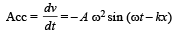∴ Acceleration (Max acc) amplitude = Aω2 = 4π2v2A

Q.2. Sound waves of frequency 660 Hz fall normally on a perfectly reflecting wall. The shortest distance from the wall at which the air particles have maximum amplitude of vibration is ..... metres.      (1984- 2 Marks)

Ans. 0.125 m

Solution.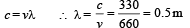The rarefaction will be at a distance of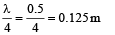Q.3. Two simple harmonic motions are represented by the equations

y1 = 10 sin (3πt +π / 4) and y2 = 5(sin 3πt + √3 cos 3πt)

Their amplitudes are in the ratio of ..........      (1986 - 2 Marks)

Ans. 1 : 1

Solution.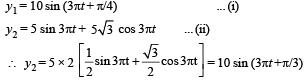The ratio of amplitudes is  10 : 10 = 1 : 1

Q.4. In a sonometer wire, the tension is maintained by suspending a 50.7 kg mass from the free end of the wire. The suspended mass has a volume of 0.0075 m3 . The fundamental frequency of vibration of the wire is 260 Hz. If the suspended mass is completely submerged in water, the fundamental frequency will become .............Hz. (1987 - 2 Marks)

Ans. 240 Hz

Solution.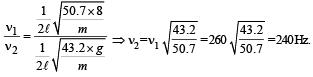Q.5. The amplitude of a wave disturbance propagating in the positive x-direction is given by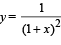at time t = 0 and by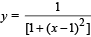at t = 2 seconds, where x and y arein metres. The shape of the wave disturbance does not change during the propagation. The velocity of the wave is .............. m/s. (1990 - 2 Marks)

Ans. 0.5 ms–1

Solution.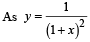At t = 0 and x = 0, we get y = 1.

Also at t = 2 and x = 1, again y = 1

The wave pulse has travelled a distance of 1m in 2 sec.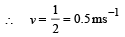Q.6. A cylinder resonance tube open at both ends has fundamental frequency F in air. Half of the length of the tube is dipped vertically in water. The fundamental frequency to the air column now is ....................       (1992 - 1 Mark)

Ans. f

Solution.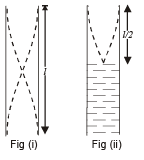In figure (i)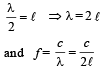In figure (ii)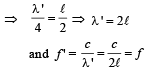Q.7. A bus is moving towards a huge wall with a velocity of 5 ms–1. The driver sounds a horn of frequency 200 Hz. The frequency of the beats heard by a passenger of the bus will be..... Hz (Speed of sound in air = 342 ms–1)         (1994 - 2 Marks)

Ans. 6 Hz

Solution. The first frequency that driver of bus hears is the original frequency of 200 Hz. The second frequency that driver hears is the frequency of sound reflected from the wall. The two frequencies of sound heard by driver is
(a) Original frequency (200 Hz.)
(b) Frequency of sound reflected from the wall (n')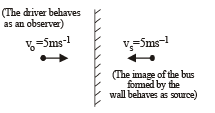The frequency of sound reflected from the wall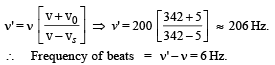True/False

Q.1. A man stands on the  ground at a fixed distance from a siren which emits sound of fixed amplitude. The man hears the sound to be louder on a clear night than on a clear day.      (1980)

Ans. F

Solution. The intensity of sound at a given point is the energy per second received by a unit area perpendicular to the direction of propagation.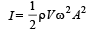Also intensity varies as distance from the point source as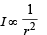NOTE : None of the parameters are changing in case of a clear night or a clear day.

Therefore the intensity will remain the same.

Q.2. A plane wave of sound travelling in air is incident upon a plane water surface. The angle of incidence is 60°. Assuming snell’s law to be valid for sound waves, it follows that the sound wave will be refracted into water away from the normal.     (1984- 2 Marks)

Ans. T

Solution. Speed of sound waves in water is greater than in air.

Q.3. A source of sound with frequency 256 Hz is moving with a velocity V towards a wall and an observer is stationary between the source and the wall. When the observer is between the source and the wall he will hear beats     (1985 - 3 Marks)

Ans. F

Solution. NOTE : If the sound reaches the observer after being reflected from a stationary surface and the medium is also stationary, the image of the source will become the source of reflected sound.

Thus in both the cases, one sound coming directly from the source and the other coming after reflection will have the same apparent frequency (Since velocity of source w.r.t. observer is same in both the cases). Therefore no beats will be heard.

The document Fill in the Blanks: Waves | JEE Advanced Notes | Study Physics 35 Years JEE Main & Advanced Past year Papers - JEE is a part of the JEE Course Physics 35 Years JEE Main & Advanced Past year Papers.
All you need of JEE at this link: JEEUse Code STAYHOME200 and get INR 200 additional OFF Use Coupon Code

Top Courses for JEEPhysics 35 Years JEE Main & Advanced Past year Papers

88 docs|49 tests

Top Courses for JEETrack your progress, build streaks, highlight & save important lessons and more!

,

,

,

,

,

,

,

,

,

,

,

,

,

,

,

,

,

,

,

,

,

;